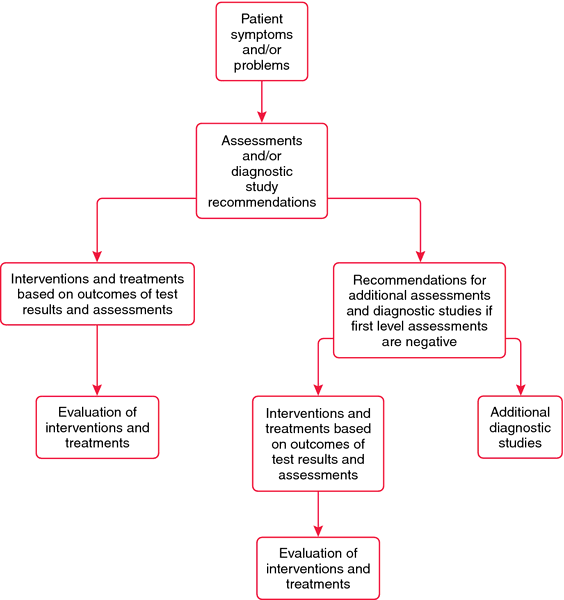# algorithm

(redirected from ALGO)
Also found in: Dictionary, Thesaurus, Financial, Acronyms, Encyclopedia, Wikipedia.

## algorithm

[al´go-rithm]
1. a series of algebraic equations.
2. a logical progression that is programmed for a computer.
3. a model for making decisions.Algorithm. Model of a decision algorithm. ACC/AHA Guidelines for the Management of Patients with Unstable Angina and Non-ST Segment Elevation Myocardial Infarction. JACC 2000, 36: 970-1062. Copyright 2000, by the American College of Cardiology and American Heart Association. Permission granted for one time use. Further reproduction is not permitted without permission of the ACC/AHA.

## al·go·rithm

(al'gō-ridhm),
A systematic process consisting of an ordered sequence of steps, each step depending on the outcome of the previous one. In clinical medicine, a step-by-step protocol for management of a health care problem; in computed tomography, the formulas used to calculate the final image from the transmitted x-ray data.
[Mediev. L. algorismus, after Muhammad ibn-Musa al-Khwarizmi, Arabian mathematician, + G. arithmos, number]

## algorithm

/al·go·rithm/ (al´go-rith'm)
1. a step-by-step method of solving a problem or making decisions, as in making a diagnosis.
2. an established mechanical procedure for solving certain mathematical problems.

## algorithm

[al′gərith′əm]
1 a step-by-step procedure for the solution to a problem by a computer, using specific mathematical or logical operations. Compare heuristic.
2 an explicit protocol with well-defined rules to be followed in solving a health care problem.

## algorithm

(1) A sequential procedure for solving a mathematical problem.
(2) A step-by-step procedure for reaching a decision when choosing among multiple alternative options, linked to each other by a decision tree.

## algorithm

Decision-making A logical set of rules for solving a specific problem, which assumes that all of the data is objective, that there are a finite number of solutions to the problem, and that there are logical steps that must be performed to arrive at each of those solutions NIHspeak A step-by-step procedure for solving a problem; a formula. See Back-propagation, Critical pathway, Genetic algorithm, Risk of ovarian cancer algorithm.

## al·go·rithm

(al'gŏr-idhm)
1. A process consisting of steps, each depending on the outcome of the previous one.
2. clinical medicine A step-by-step protocol for management of a health care problem.
3. computed tomography The formulas used for calculation of the final image from the x-ray transmission data.
[Mediev. L. algorismus, after Muhammad ibn-Musa al-Khwarizmi, Persian mathematician, + G. arithmos, number]

## al·go·rithm

(al'gŏr-idhm)
A systematic process consisting of an ordered sequence of steps, each step depending on the outcome of the previous one.
[Mediev. L. algorismus, after Muhammad ibn-Musa al-Khwarizmi, Persian mathematician, + G. arithmos, number]

## algorithm

a set of rules designed to solve a specific problem by proceeding through a series of prearranged, logical steps. Originally referred to purely mathematical problems, now used in a wider sphere, e.g. to solve diagnostic problems. Often depicted in the form of a box and line diagram which sets out the logic of the procedure or program.

diagnostic algorithm
mapping of the logical steps to be taken in eliminating potential diagnoses which do not match clinical signs or pathological findings and arranging possible diagnoses in order of probability.
References in periodicals archive ?
The other difference between futures and equities algos is the thorny issue of expiry.
This combined offering will enable Compositedge clients to develop, test and deploy their algos seamlessly.
This combined offering will enable Destimoney to develop, test and deploy their algos seamlessly.
Institutional Traders in the advanced capital markets have traditionally used conventional execution algos like VWAP, TWAP, POV etc.

Site: Follow: Share:
Open / Close